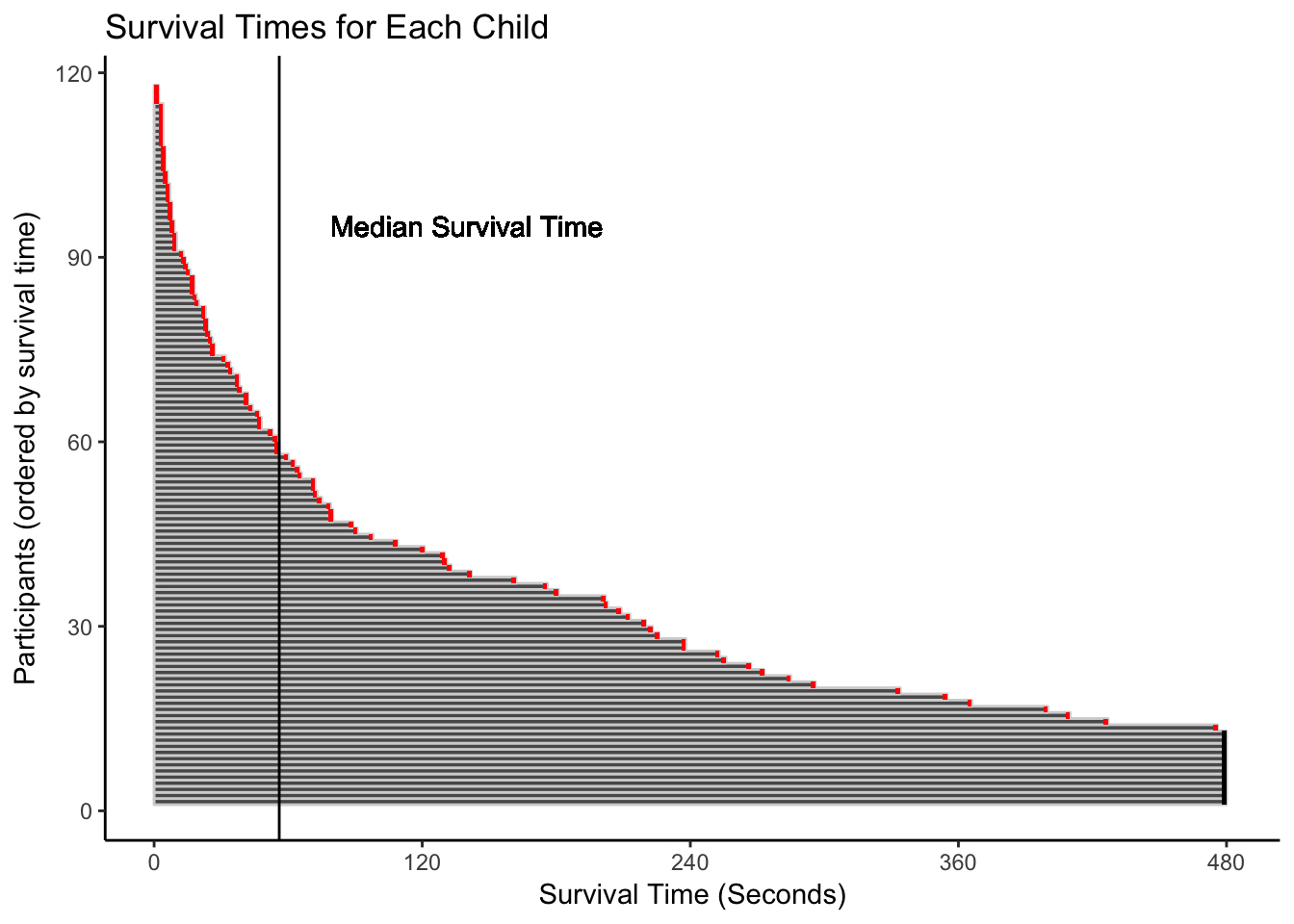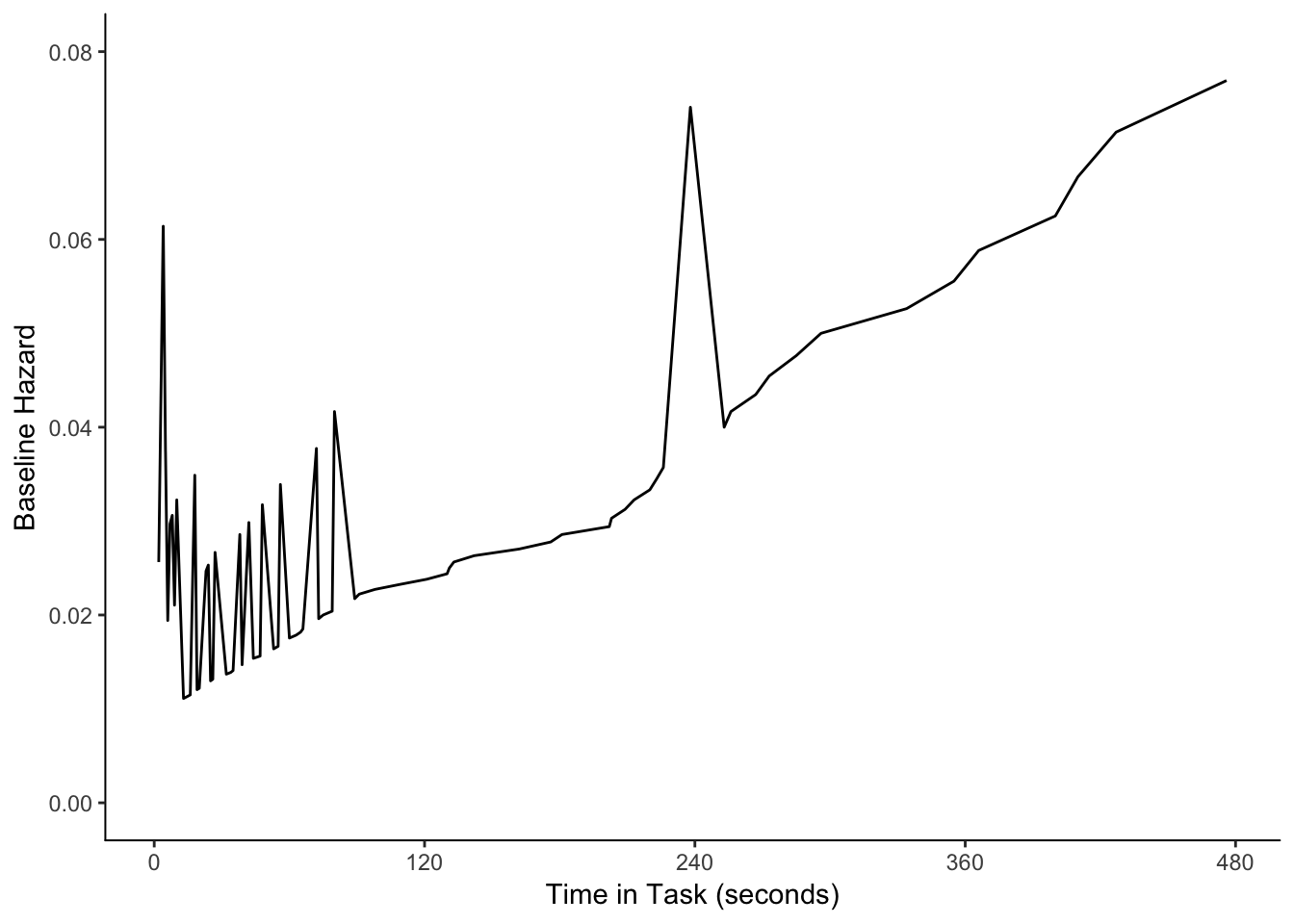# Overview

This tutorial is Part 2 of 5 showing how to do survival analysis with observational data (video recordings of participant behavior), using data from a study of children’s emotion regulation as an example (Cole et al., 2011). In this tutorial, we will demonstrate how to conduct a single episode model with time-invariant predictors. With this model, we examine interindividual differences in children’s negative affectivity, to test if (a) children higher in negative affectivity also expressed anger for the first time earlier in the wait task, and (b) children lower in negative affectivity also took longer to express anger for the first time.

## Set-up

Set working directory and read in data

setwd("~/Desktop/Data/Survival analysis")

names(wait36)
##  "id"                     "status"
##  "event_time"             "negative_affectivity30"

library(ggplot2)
library(psych)
library(survival)
library(eha)
library(reshape)

Subset to relevant variables:

wait36a <-wait36
head(wait36a)
##   id status event_time negative_affectivity30
## 1  1      1         39               2.793750
## 2  2      1        203               3.614583
## 3  3      1         80               4.347222
## 4  5      1         10               3.745960
## 5  6      1         14               3.933523
## 6  8      1         89               3.043750

## Outline

• Step 1: Preliminary Considerations
• Step 2: Data Preparation
• Step 3: Data Description
• Step 4: Model Building and Assessment of Fit to the Data
• Step 5: Presentation and Interpretation of Results

## Step 1: Preliminary Considerations

It is important to first determine the kind of model that will be used to answer research questions, as other steps (e.g., data preparation) will depend on what type of model will be fit. Specifically, researchers need to decide whether models will:

• Be fit in discrete or continuous time
• Include single or repeating occurrences of the dependent variable
• Be fit with a non-parametric, semi-parametric, or parametric approach
• Include predictors that are time invariant or time varying (or both)

With this model, we examine interindividual differences in children’s negative affectivity, to test if (a) children higher in negative affectivity also expressed anger for the first time earlier in the wait task, and (b) children lower in negative affectivity also took longer to express anger for the first time. We will use a semi-parametric approach (Cox regression), because we do not know the shape of the underlying distribution (which precludes the use of a parametric approach) and because we want to incorporate multiple predictors as time-invariant predictors (which is for several reasons not ideal with a non-parametric approach).

## Step 2: Data Preparation

See Part 1 for data preparation steps

## Step 3: Data Description

Now, we need to examine several characteristics of the data before we start modeling. Specifically, we need to examine:

• How many cases in the data are right-censored. We already examined left-censoring in Step 2 so we do not need to examine that further in Step 3. If there are a high number of right-censored cases, we will need to examine if right-censored cases differ from non-censored cases in systematic ways (e.g., by predictors to be used in model).
• How many ties (cases with exactly the same survival time) are present in the data. If a researcher has data with continuous time, a large number of ties could be problematic but this is unlikely with observational data. If a large number of ties are present, the researcher could aggregate time into discrete values and use a discrete-time model to overcome the presence of many ties.
• The median survival time. Standard descriptive statistics (mean, standard deviation) will not provide accurate information about survival analysis data because of censoring. The median survival time is used instead.
• A plot of survival times to understand how survival times are distributed in the data.
• Descriptive statistics for any predictors in the model. We will be looking at negative affectivity.

### Determine how much of sample has right censoring

Some kids in these data are right-censored such that the period of observation expired before they expressed anger.

length(wait36a[ which(wait36a$status==0),]$id)
##  12

In this case, 12 of the 117 children in the sample have right censoring, which is within the acceptable range.

### Determine how much of sample has left censoring

See sections in step 2 (data preparation, Part 1) for this information. In these data, 7 cases were left censored, meaning that 7 children were expressing anger at the start of the observation. To overcome the issue of left censoring, we defined the start of the observation at the first second of the wait task during which children were not expressing anger.

table(wait36a$event_time) ## ## 2 4 5 6 7 8 9 10 13 14 15 16 18 19 20 23 24 25 ## 3 7 4 2 3 3 2 3 1 1 1 1 3 1 1 2 2 1 ## 26 27 32 34 35 38 39 42 44 47 48 53 55 56 60 63 65 66 ## 1 2 1 1 1 2 1 2 1 1 2 1 1 2 1 1 1 1 ## 72 73 75 79 80 89 91 98 109 121 130 131 133 142 162 176 181 202 ## 2 1 1 1 2 1 1 1 1 1 1 1 1 1 1 1 1 1 ## 203 209 213 220 223 226 238 253 256 267 273 285 296 334 355 366 400 410 ## 1 1 1 1 1 1 2 1 1 1 1 1 1 1 1 1 1 1 ## 427 476 480 ## 1 1 12 ### Calculate the median survival time summary(wait36a$event_time)
##    Min. 1st Qu.  Median    Mean 3rd Qu.    Max.
##     2.0    15.0    56.0   134.1   220.0   480.0

The median survival time is 56 seconds, indicating that half of the children had expressed anger for the first time by 56 seconds into the task.

### Plot survival times and ties

Create new incremental count id:

wait36a$idcount <- c(1:length(wait36a$id))

Order by survival time and create an order variable:

wait36a <- wait36a[order(-wait36a$event_time,wait36a$status),]
wait36a$order <- c(1:length(wait36a$id))

Create an ordered survival time plot:

ggplot(data=wait36a, aes(x=event_time, y=order)) +
geom_rect(xmin=0,xmax=wait36a$event_time,ymin=wait36a$order,ymax=wait36a$order+1, colour="lightgray") + geom_rect(xmin=wait36a$event_time-2,xmax=wait36a$event_time,ymin=wait36a$order,ymax=wait36a$order+1, fill=factor(wait36a$status+1)) +
geom_vline(xintercept= 56,linetype="solid") +
scale_x_continuous(breaks=seq(0,480,120)) +
geom_text(aes(140, 95, label="Median Survival Time")) +
xlab("Survival Time (Seconds)") + ylab("Participants (ordered by survival time)") +
ggtitle("Survival Times for Each Child") +
theme_classic() +
theme(legend.position="none",
panel.grid.major=element_blank(),
panel.grid.minor=element_blank(),
panel.background=element_blank(),
axis.line.x = element_line(color = "black"),
axis.line.y = element_line(color = "black"))### Examine descriptive statistics of predictors

Center predictors:

wait36a$negative_affectivity30.c <- scale(wait36a$negative_affectivity30, center=TRUE, scale=FALSE)

#Multiply by 100 to make interpretation easier, centered for inclusion in model:
wait36a$negative_affectivity30.c100 <- wait36a$negative_affectivity30.c*100

#And uncentered for reporting:
wait36a$negative_affectivity30.100 <- wait36a$negative_affectivity30*100

Decribe model predictors and make sure transformations look good:

describe(wait36a[,c("negative_affectivity30")])
##    vars   n mean   sd median trimmed  mad  min  max range skew kurtosis
## X1    1 112 3.56 0.55    3.6    3.55 0.52 2.27 4.96  2.68 0.07    -0.33
##      se
## X1 0.05
describe(wait36a[,c("negative_affectivity30.100")])
##    vars   n   mean    sd median trimmed   mad    min    max  range skew
## X1    1 112 355.93 54.55 360.47   355.4 51.69 227.22 495.58 268.36 0.07
##    kurtosis   se
## X1    -0.33 5.15

## Step 4: Model Building, Estimation, and Assessment of Fit to the Data

We will now run our Cox regression models. First, we will fit a baseline model (intercept only; no predictors included) to examine the survival and cumulative hazard functions. Next, we will include negative affectivity as a predictor to test our research question. Then, we will use diagnostics to examine model fit.

### Cox Regression Model

The equation for the model is specified as,

$$h_{i} = h_{0} exp(\beta_{1}NegativeAffectivity_{i})$$

### Fit Cox Regression Model - Baseline

Fit baseline/null model (no predictors) using the survival package:

coxmodel1 <- coxph(formula = Surv(event_time, status) ~ 1,
data = wait36a)
summary(coxmodel1)
## Call:  coxph(formula = Surv(event_time, status) ~ 1, data = wait36a)
##
## Null model
##   log likelihood= -423.4879
##   n= 117
model1fit <- survfit(coxmodel1)

Fit baseline/null model (no predictors) using the eha package. We need to do this step in this package in order to plot the non-cumulative hazard function.

coxmodel1b <- coxreg(formula = Surv(event_time, status) ~ 1,
data = wait36a,center=FALSE)
summary(coxmodel1b)
## Call:
## coxreg(formula = Surv(event_time, status) ~ 1, data = wait36a,
##     center = FALSE)
##
## Null model
## NULL

### Plot the Non-cumulative Hazard Function

Get $$H_0$$ :

model1bdata <- data.frame(matrix(unlist(coxmodel1b$hazards),ncol=2,nrow=74)) colnames(model1bdata) <- c("time","basehaz") Plot: ggplot(model1bdata,(aes(x=time,y=basehaz))) + geom_line() + scale_x_continuous(breaks=seq(0,480,120)) + scale_y_continuous(breaks=seq(0,0.08,.02)) + expand_limits(y=c(0,0.08)) + xlab("Time in Task (seconds)") + ylab("Baseline Hazard") + theme(panel.background=element_blank(), axis.line.x = element_line(color = "black"), axis.line.y = element_line(color = "black"))### Data Set-up Create a dataframe with baseline model information so we can plot baseline survival and hazard functions: model1data <- data.frame(cbind(model1fit$time,model1fit$surv,model1fit$cumhaz,model1fit$upper,model1fit$lower))
names(model1data) <- c("time","surv","cumhaz","upperCI","lowerCI")

### Plot the Cumulative Hazard Function

ggplot(model1data,aes(x=time)) +
geom_line(aes(y=cumhaz),size=1) +
scale_x_continuous(breaks=seq(0,480,120)) +
scale_y_continuous(breaks=seq(0,2.5,.5)) +
expand_limits(y=c(0,2.5)) +
xlab("Time in Task (seconds)") + ylab("Cumulative Hazard Function") +
ggtitle("Cumulative Hazard Function for First Anger") +
theme(panel.background=element_blank(),
axis.line.x = element_line(color = "black"),
axis.line.y = element_line(color = "black"))